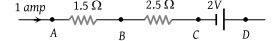NEET Physics Current Electricity Questions Solved

In the circuit element given here, if the potential at point B, VB = 0, then the potentials of A and D are given as(1) ${V}_{A}=-1.5\text{\hspace{0.17em}}V,\text{\hspace{0.17em}}{V}_{D}=+2\text{\hspace{0.17em}}V$

(2) ${V}_{A}=+1.5\text{\hspace{0.17em}}V,\text{\hspace{0.17em}}{V}_{D}=+2\text{\hspace{0.17em}}V$

(3) ${V}_{A}=+1.5\text{\hspace{0.17em}}V,\text{\hspace{0.17em}}{V}_{D}=+0.5\text{\hspace{0.17em}}V$

(4) ${V}_{A}=+1.5\text{\hspace{0.17em}}V,\text{\hspace{0.17em}}{V}_{D}=-0.5\text{\hspace{0.17em}}V$

Explanation is a part of a Paid Course. To view Explanation Please buy the course.

Difficulty Level: